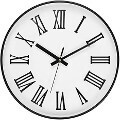# Roman numerals calculatorRoman numerals are based on seven symbols (IVXLCDM). A smaller number in front of a larger number means subtraction, all else means addition. For example, IV means 4, VI means 6.

You would not put more than one smaller number in front of a larger number to subtract. For example, IIV would not mean 3.

You must separate ones, tens, hundreds, and thousands as separate items. That means that 99 is XCIX, 90 + 9, but never should be written as IC. Similarly, 999 cannot be IM and 1999 cannot be MIM.

#### I

The numeral one. II is two, III is three.

#### V

The numeral 5. IV is 4, VI is 6, VII is 7, VIII is 8.

#### X

The numeral 10. IX is 9, XI is 11, etc.

#### L

The numeral 50. XL would be 40.

The numeral 100.

The numeral 500.

#### M

The numeral 1000.
Sometimes you will see a numeral with a line over it. That means to multiply it by 1000. A numeral V with a line over it means 5000.